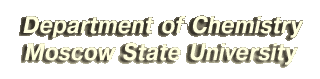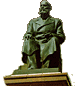# Example Problems for Entrance Examinations

## Main round

Problem 1.

Which substance can enter redox reaction with FeCl3 and exchange reaction with AgNO3? Write both reactions.
(2 points) [Solution]

Problem 2.

List the given substances in the decreasing order of their activity in electrophilic substitution (SE) reactions: chlorobenzene, toluene, nitrobenzene. Justify your answer. Write two SE reactions with the least active substance.
(2 points) [Solution]

Problem 3.

Activation energy of some reaction is twice as large as that of another reaction. Upon heating from T1 to T2, the rate constant of the first reaction increased by a factor of a. How many times would the rate constant of the second reaction increase at heating from T1 to T2?.
(2 points) [Solution]

Problem 4.

Write the reactions involved in the following sequence of transformations:Determine the functional groups X and Y. Specify the conditions of all reactions.
(3 points) [Solution]

Problem 5.

Write the reactions involved in the following sequence of oxidation number transformations:
(3 points) [Solution]

P+3S+4Ba+2C+2

Problem 6.

The 2.33 g of formaldehyde solution in a mixture of acetic and formic acids completely reacted with 18.7 ml of the 8.4% aqueous solution of potassium hydroxide (the solution density is 1.07 g/ml). The resulting solution reacted with an excess of water-ammonia solution of silver nitrate with the formation of 9.72 g of a precipitate. Determine the molar fractions of components in the initial solution.
(4 points) [Solution]

Problem 7.

Gas obtained by acting of an excess of concentrated nitric acid on 4.8 g of copper was passed through 100 g of 10% aqueous solution of sodium hydroxide. Determine the maximum amount of iodine that can be obtained by adding potassium iodide to the resulting solution.
(4 points) [Solution]

• Example Problems in Chemistry for the Entrance Exams (preliminary round)
• Special Program developed for chemistry entrance examinations in Moscow State University.

Chemistry Department of Moscow State UniversityThe site is supported by Russian Foundation for Basic Research
The using of published on this page materials is not allowed without special permission
Copyright (C) Chemisty Department of Moscow State University
Web-Editor: B.I.Pokrovskii
Web-design: Copyright (C) I. Minyaylova and V. Minaylov
webmaster@www.chem.msu.su